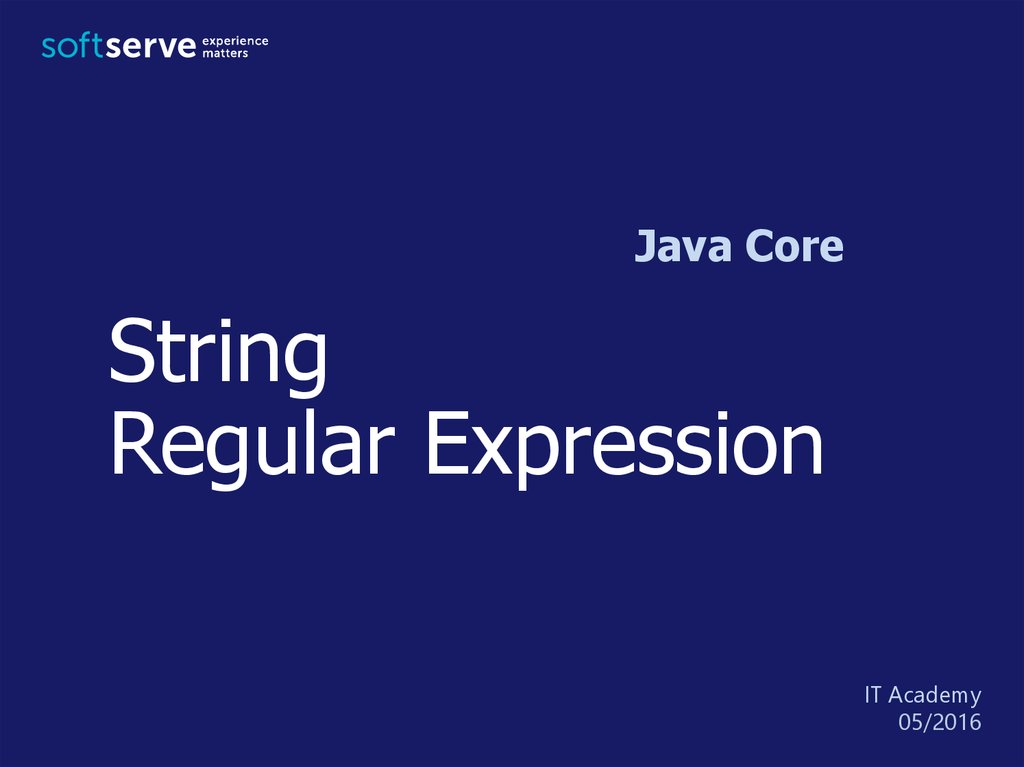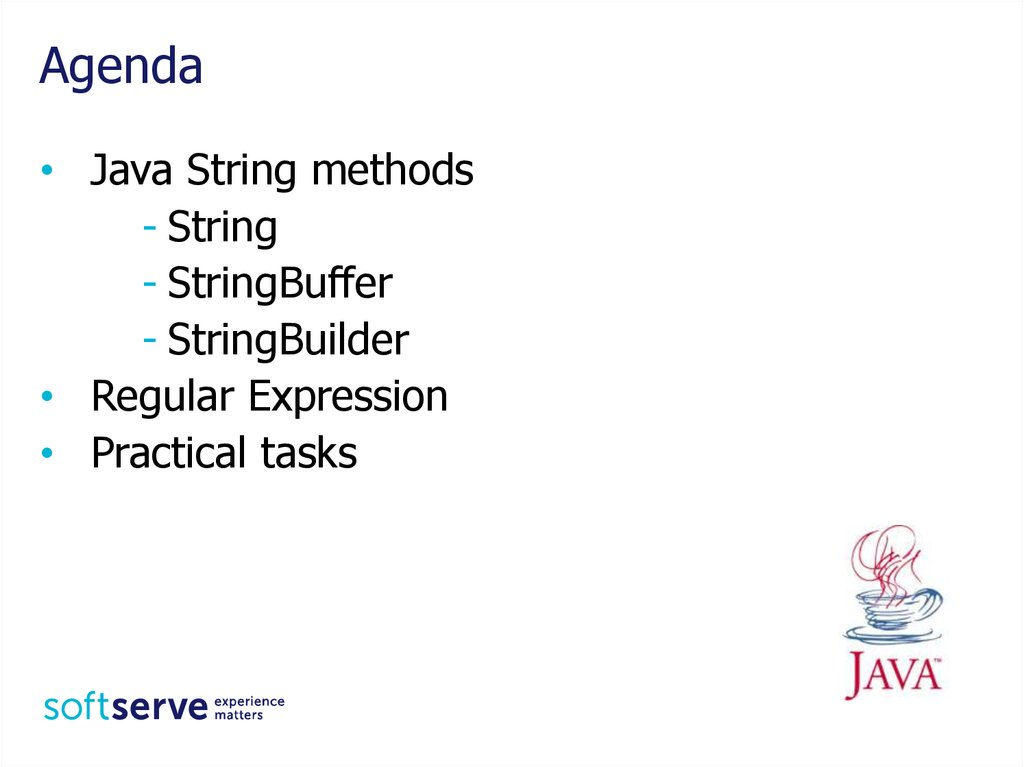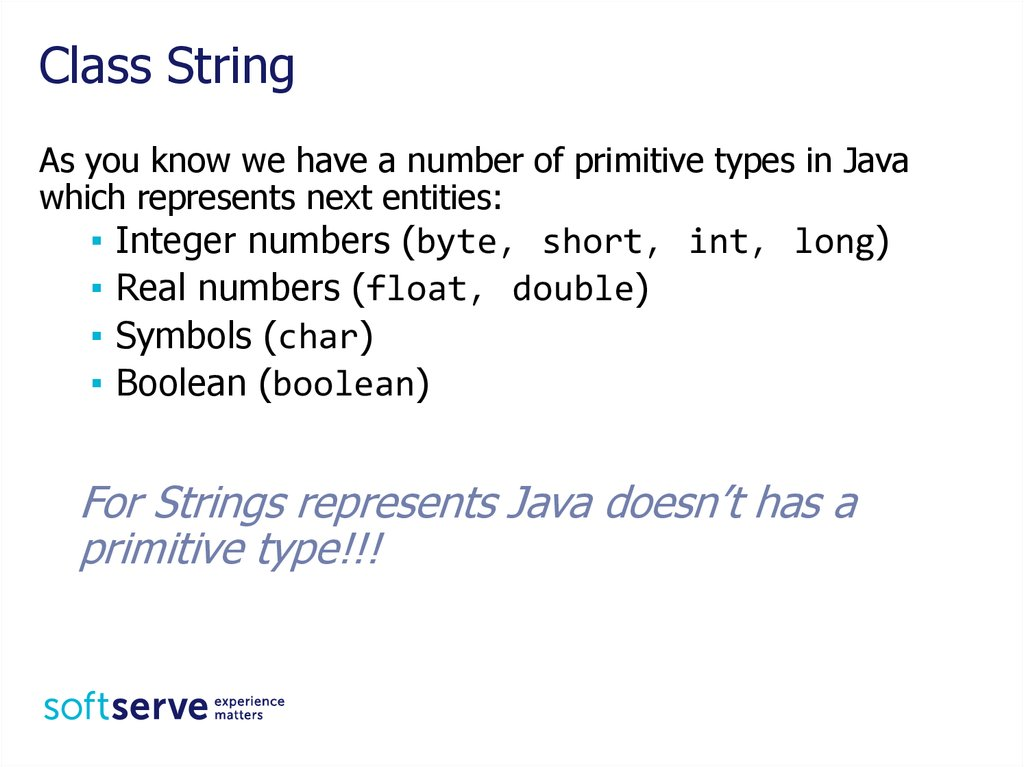# String Regular Expression. Java Core

## 1. String Regular Expression

Java Core
String
Regular Expression
05/2016

## 2. Agenda

• Java String methods
- String
- StringBuffer
- StringBuilder
• Regular Expression

## 3. Class String

As you know we have a number of primitive types in Java
which represents next entities:

Integer numbers (byte, short, int, long)
Real numbers (float, double)
Symbols (char)
Boolean (boolean)
For Strings represents Java doesn’t has a
primitive type!!!

## 4. String

• Strings, which are widely used in Java programming, are a
sequence of characters.
• In the Java programming language, strings are objects.
• The Java platform provides the String class to create and
manipulate strings.
• Literal automatically creates an object of type String
String s1 = "sun.com";
String s2 = new String("sun.com");
• String objects are immutable.
• After creating the content can not be changed.
• You can always create a new string that contains all
changes.

## 5. String

String class supports multiple constructors
- String( );
- String(StringBuffer sbuf);
- String(String str); - String(StringBuilder sbuild);
- String(char[ ] unicodechar); ...
▪ Just assignee value to variable
String strFirst = "First String";
▪ Call constructor of String class
String strSecond = new String("Second String");
▪ Call constructor of String class
char[] chA = {’A’, ’B’, ’C’, ’D’, ’E’, ’F’};
String strThird = new String(chA);
String strFourth = new String(chA, 2, 4); // CDEF

## 6. Basic methods

• Concat strings
String concat(String s) or "+"
String str1 = "Hello ";
String str2 = "World!";
String str3 = str1 + str2;
String str4 = str1.concat(str2);
System.out.println(str3 + str4);
• Get length of string
int length()
// str3Length = 12
int str3Length = str3.length();

## 7. Basic methods

• Compare strings
boolean equals(Object obj)
boolean equalsIgnoreCase(String s)
int compareTo(String s)
int compareToIgnoreCase(String s)
boolean contentEquals(StringBuffer obj)
String a = "a";
What will be the results?
String A = "A";
String b = "a";
System.out.println(a.equals(A));
System.out.println(a.equals(b));
System.out.println(a.equalsIgnoreCase(A));
System.out.println(a.compareTo(A));
System.out.println(a.compareToIgnoreCase(A));
System.out.println(a.contentEquals(A));

## 8. Basic methods

• Get part of string
- extract a substring of length m-n, starting at position n
String substring(int n, int m)
- extract a substring starting at position n
String substring(int n)
String str =
int indexOf(char ch)
"I study Java language";
boolean startsWith(String s) int n = str.indexOf('J'); //8
boolean endsWith(String s)
char c = str.charAt(8);
//J
char charAt(int position)
String str1 = str.substring(13);
String str2 = str.substring(8, 12);
// language
//Java
Boolean res = str.startsWith("I study"); //true
res = str.startsWith("Java", 8);
//true
res = str.endsWith("I study");
//false

## 9. Basic methods

• Working with case of symbols
String toLowerCase()
String toUpperCase()
• Trim strings
String str = "\tTabulated String\t";
String tStr = str.trim();
• Replace symbols
String rStr = str.replace('a', 'o');
boolean isEmpty()

## 10. Java String methods

public static void main(String[] args) {
String s1 = "Java";
String s2 = "Java";
String s3 = new String("Java");
System.out.println(s1 + "==" + s2 + " :
+ (s1 == s2));
System.out.println(s1 + "==" + s3 + " :
+ (s1 == s3));
System.out.println(s1 + " equals " + s2
+ s1.equals(s2));
System.out.println(s1 + " equals " + s3
+ s1.equals(s3));
System.out.println(s1.hashCode());
}
"
// true
"
//
+ "
//
+ "
//
false
: "
true
: "
true

## 12. Example

public static void main(String[ ] args) {
int i;
char s[ ] = { 'J', 'a', 'v', 'a' };
String str = new String(s);
// str = "Java"
if (!str.isEmpty( )) {
i = str.length( );
// i = 4
str = str.toUpperCase( );
// str = "JAVA"
String num = String.valueOf(8);
// num = "8"
num = str.concat("-" + num); // num = "JAVA-8"
char ch = str.charAt(2);
// ch = 'V'

## 13. Example

i = str.lastIndexOf('A');
// i = 3 or -1
num = num.replace("8","SE"); // num = "JAVA-SE"
str.substring(0, 4).toLowerCase( ); // java
str = num + "-8";
// str = "JAVA-SE-8"
String[ ] arr = str.split("-");
for (String s : arr) {
System.out.println(s);
}
}
}

## 14. String Formatting

System.out.printf(“format-string” [, arg1, arg2, … ] );
Format String:
% [flags] [width] [.precision] conversion-character
Flags: ‘-’ (align), ‘+’ (sign), 0 (forces zero), ‘,’ ‘ ‘ (space)
Width - minimum number of characters to be written to the output.
Precision - the number of digits of precision when outputting floating-point
values or the length of a substring to extract from a String.
Conversion-Characters:
- d : decimal integer [byte, short, int, long]
- f : floating-point number [float, double]
- c : character Capital C will uppercase the letter
- s : String Capital S will uppercase all the letters in the string
- h : hashcode A hashcode is like an address.
- n : newline use %n instead of \n

## 15. String Formatting

Supported by String.format() and System.out.printf()
methods:
public class StringSamples {
public static void main(String... args) {
final double PI = 3.1415926;
String format = "%.2f";
String s = String.format(format, PI);
System.out.println(s);
System.out.printf(format, PI);
}
}
Detailed tutorial with samples:
https://examples.javacodegeeks.com/corejava/lang/string/java-string-formatexample/

## 16. StringBuilder and StringBuffer

• String objects are immutable
• Defined equal classes StringBuffer and StringBilder allow changes
to lines
• StringBuffer is synchronized, StringBuilder is not.
String s1 = new String("Hello");
String s2 = "And Goodbye";
String str = s1 + s2;
str = s1.concat(s2);
StringBuilder sb =
new StringBuilder(s1);
sb.append(s2);
str = sb.toString( );
StringBuffer sa =
new StringBuffer( );
sa.append(s1);
sa.append(s2);
String str = sa.toString( );

## 17. StringBuilder

Constructors
• StringBuilder()
• StringBuilder(char[] seq)
• StringBuilder(int capacity)
• StringBuilder(String str)
Methods
• append(...) adds a string to the end of the buffer.
• insert(...) adds a string to any location (insert the
substring).
• delete(int begin, int end) deletes a sequence of
characters.
• int capacity() returns the current capacity of the buffer.
• void ensureCapacity(int i) changes the value of
capacity
• reverse() causes this character sequence to be replaced by
the reverse of the sequence

## 18. Regular Expression

A regular expression is a kind of pattern that can be applied to
text (Strings, in Java)
A regular expression either matches the text (or part of the
text), or it fails to match
If a regular expression matches a part of the text, then you
can easily find out which part
- Beginning with Java 1.4, Java has a regular expression
package, java.util.regex
The regular expression "[a-z]+" will match a sequence of one
or more lowercase letters
- [a-z] means any character from a through z, inclusive
- + means “one or more”

## 19. Regular Expression

Suppose we apply this pattern to the String
"Now is the time"
First, you must compile the pattern
import java.util.regex.*;
Pattern p = Pattern.compile("[a-z]+");
Next, you must create a matcher for a specific piece of text by
sending a message to your pattern
Matcher m = p.matcher("Now is the time");
Neither Pattern nor Matcher has a public constructor; you
create these by using methods in the Pattern class

## 20. Regular Expression

Now that we have a matcher m:
• m.matches() returns true if the pattern matches the entire
text string, and false otherwise
• m.lookingAt() returns true if the pattern matches at the
beginning of the text string, and false otherwise
• m.find() returns true if the pattern matches any part of
the text string, and false otherwise
If called again, m.find() will start searching from where
the last match was found
m.find() will return true for as many matches as there
are in the string; after that, it will return false
When m.find() returns false, matcher m will be reset to
the beginning of the text string (and may be used again)

## 21. Regular Expression

import java.util.regex.*;
public class Appl {
public static void main(String[] args) {
String pattern = "[a-z]+";
String text = "Now is the time";
Pattern p = Pattern.compile(pattern);
Matcher m = p.matcher(text);
while (m.find()) {
System.out.print(text
.substring(m.start(), m.end()) + "*");
}
}

## 22. Regular Expression

abc
exactly this sequence of three letter
[abc]
any one of the letters a, b, or c
[^abc]
any character except one of the letters a, b, or c
(immediately within an open bracket, ^ mean
“not,” but anywhere else it just means the
character ^)
[a-z]
any one character from a through z, inclusive
[a-zA-Z0-9] any one letter or digit

## 23. Regular Expression

If one pattern is followed by another, the two patterns must
match consecutively
- For example, [A-Za-z]+[0-9] will match one or more letters
immediately followed by one digit
- The vertical bar, |, is used to separate alternatives
- For example, the pattern abc|xyz will match either abc or xyz
X?
X*
X+
X{n}
X{n,}
X{n, m}
optional, X occurs once or not at all
X occurs zero or more times
X occurs one or more times
X occurs exactly n times
X occurs n or more times
X occurs at least n but not more than m times

## 24. Regular Expression

.
\d
\D
\s
\S
\w
\W
^
\$
\b
\B
any one character except a line terminator
a digit: [0-9]
a non-digit: [^0-9]
a whitespace character: [ \t\n\x0B\f\r]
a non-whitespace character: [^\s]
a word character: [a-zA-Z_0-9]
a non-word character: [^\w]
the beginning of a line
the end of a line
a word boundary
not a word boundary

## 25. Regular Expression

In some implementations, a quantifier in regular expressions
corresponds to the maximum line length is possible
For example, often expect that the expression (<.*>) will be
found in the text tag HTML. However, if the text is more than
one HTML-tag, this expression matches the entire string
containing a set of tags.
<p><b>Beginning with bold text</b> next,
text body,<i>italic text</i> end of text.</p>
Solved problem:
- Take into account characters that are not relevant to the
desired pattern (<[^>]*> for the above case)

## 26. Regular Expression

import java.util.regex.*;
public class Appl {
public static void main(String[] args) {
//String pattern = "[a-z]+";
//String text = "Now is the time";
//
//String pattern = "<.*>";
//String pattern = "<[^>]*>";
//String text = "<p><b>Beginning with bold text</b>
next, text body,<i>italic text</i> end of text.</p>";
String pattern = "\\w+(\\.\\w+)*@(\\w+\\.)+\\w+";
String text = "my.m[email protected]";

## 27. Regular Expression

Pattern p = Pattern.compile(pattern);
Matcher m = p.matcher(text);
if (m.matches()) {
System.out.print("Matches the entire text string");
m.reset();
System.out.println();
}
while (m.find()) {
System.out.print(text.substring(m.start(),m.end())+ "*");
}
}
}

1. Enter the two variables of type String. Determine whether the
first variable substring second. For example, if you typed «IT»
2. Enter surname, name and patronymic on the console as a
variable of type String. Output on the console:
• surnames and initials
• name
• name, middle name and last name
3. The user name can be 3 to 15 characters of the Latin alphabet,
numbers, and underscores. Using regular expressions implement
checking the user name for validity. Input five names in the main
method . Output a message to the console of the validation of
each of the entered names.

## 29. Homework

1. Enter in the console sentence of five words.
• display the longest word in the sentence
• determine the number of its letters
• bring the second word in reverse order
2. Enter a sentence that contains the words between more than
one space. Convert all spaces, consecutive, one. For example, if
we introduce the sentence "I am
learning
Java Core»,
we have to get the "I'm learning Java Core»
3. Implement a pattern for US currency: the first symbol "\$", then
any number of digits, dot and two digits after the dot. Enter the
text from the console that contains several occurrences of US
currency. Display all occurrences on the screen.

## 30. The end

USA HQ
Toll Free: 866-687-3588
Tel: +1-512-516-8880
Ukraine HQ
Tel: +380-32-240-9090
Bulgaria
Tel: +359-2-902-3760
Poland
Tel: +48-71-382-2800
EMAIL
[email protected]
Germany
Tel: +49-69-2602-5857
UK
Tel: +44-207-544-8414
WEBSITE:
www.softserveinc.com
Netherlands
Tel: +31-20-262-33-23